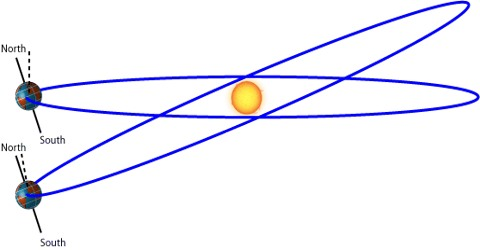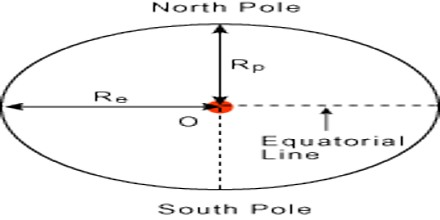# Variation due to Shape of the Earth

Variation due to shape of the earth:

We know, the shape of the earth is not exactly spherical. Its north-south is slightly squeezed and east-west is slightly flattened. That means the earth is an oblate spheroid [Fig].The polar radius of the earth is about 22 km more than that of the equatorial radius. Now, we know g at a place is inversely proportional to the square of the radius of the earth, hence the value of g at the pole is maximum and that at the equator is minimum and in any other place it is between these two terminal values.

The shape of the earth is bulged at the equator and flat at the poles. This means earth has large radius at the equator than at poles. We know that,

g = GM/R2;     re > rP

ge = GM/Re2

gp = GM/Rp2

So, gequator < gpole

So, acceleration due to gravity is more at the pole than at the equator.